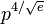# Primitive root

In number theory, a primitive root of a modulus is a number whose powers run through all the residue classes coprime to the modulus. This may be expressed by saying that n has a primitive root if the multiplicative group modulo n is cyclic, and the primitive root is a generator, having an order equal to Euler's totient function φ(n). Another way of saying that n has a primitive root is that the value of Carmichael's lambda function, λ(n) is equal to φ(n).

The numbers which possess a primitive root are:

• 2 and 4;
• pn and 2pn where p is an odd prime.

If g is a primitive root modulo an odd prime p, then one of g and g+p is a primitive root modulo p2 and indeed modulo pn for all n.

There is no known fast method for determining a primitive root modulo p, if one exists. It is known that the smallest primitive root modulo p is of the order of$p^{4/\sqrt e}$ by a result of Burgess, and if the generalised Riemann hypothesis is true, this can be improved to an upper bound of 2(logp)2 by a result of Bach.

## Artin's conjecture

Artin's conjecture for primitive roots states that any number g which is not a perfect square is infinitely often a primitive root. Roger Heath-Brown has shown that there are at most two exceptional prime numbers a for which Artin's conjecture fails.